# Dual Y axis with R and ggplot2

This post describes how to build a dual Y axis chart using `R` and `ggplot2`. It uses the `sec.axis` attribute to add the second Y axis. Note that this kind of chart has major drawbacks. Use it with care.

# Visualizing 2 series with `R` and `ggplot2`

Let’s consider a dataset with 3 columns:

• `date`
• first serie to display: fake `temperature`. Range from 0 to 10.
• second serie: fake `price`. Rangee from 0 to 100.

One could easily build 2 line charts to study the evolution of those 2 series using the code below.

But even if strongly unadvised, one sometimes wants to display both series on the same chart, thus needing a second Y axis.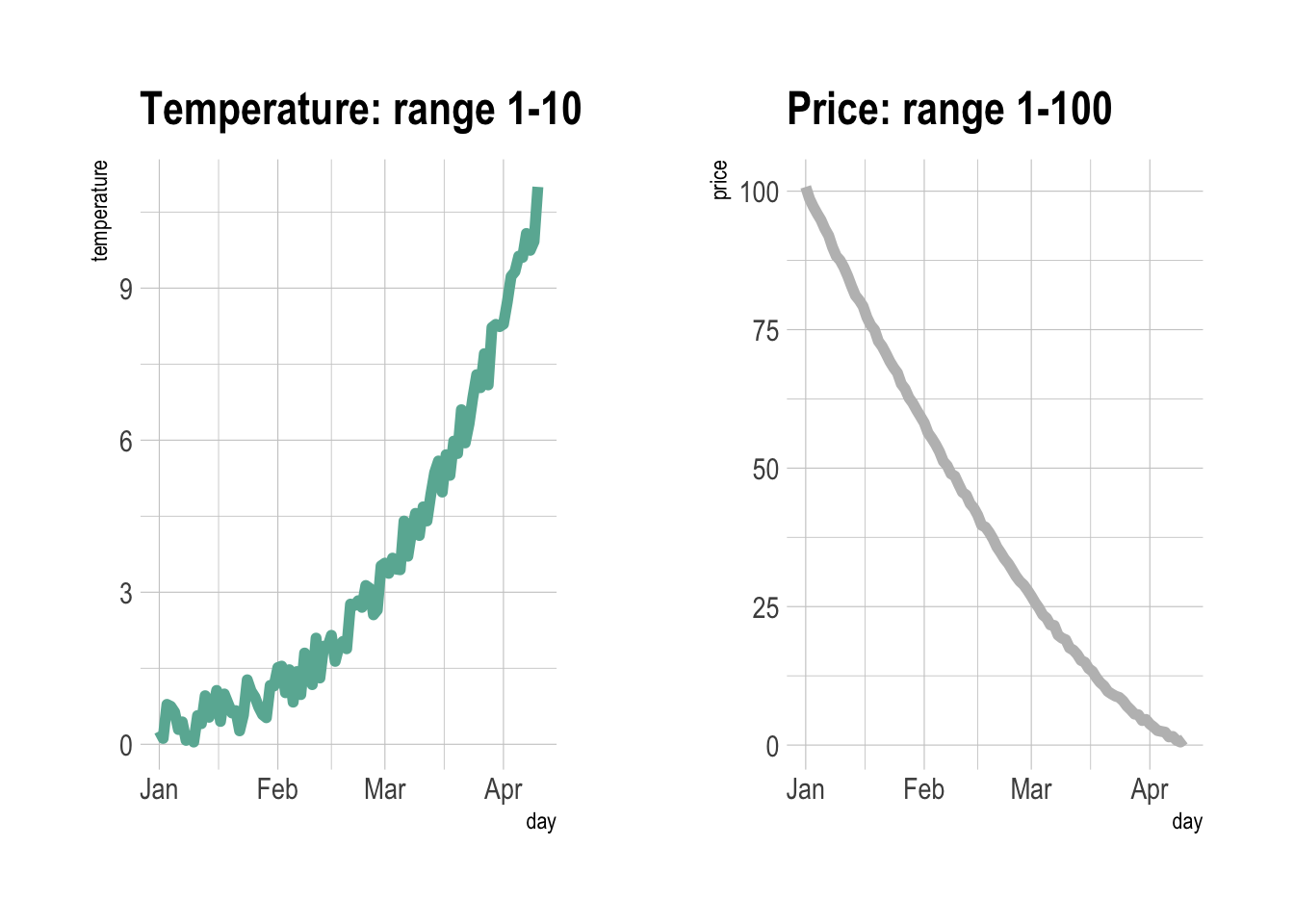``````# Libraries
library(ggplot2)
library(dplyr)
library(patchwork) # To display 2 charts together
library(hrbrthemes)

# Build dummy data
data <- data.frame(
day = as.Date("2019-01-01") + 0:99,
temperature = runif(100) + seq(1,100)^2.5 / 10000,
price = runif(100) + seq(100,1)^1.5 / 10
)

# Most basic line chart
p1 <- ggplot(data, aes(x=day, y=temperature)) +
geom_line(color="#69b3a2", size=2) +
ggtitle("Temperature: range 1-10") +
theme_ipsum()

p2 <- ggplot(data, aes(x=day, y=price)) +
geom_line(color="grey",size=2) +
ggtitle("Price: range 1-100") +
theme_ipsum()

# Display both charts side by side thanks to the patchwork package
p1 + p2``````

# Adding a second Y axis with `sec.axis()`: the idea

`sec.axis()` does not allow to build an entirely new Y axis. It just builds a second Y axis based on the first one, applying a mathematical transformation.

In the example below, the second Y axis simply represents the first one multiplied by 10, thanks to the `trans` argument that provides the `~.*10` mathematical statement.

Note that because of that you can’t easily control the second axis lower and upper boundaries. We’ll see a trick below in the tweaking section.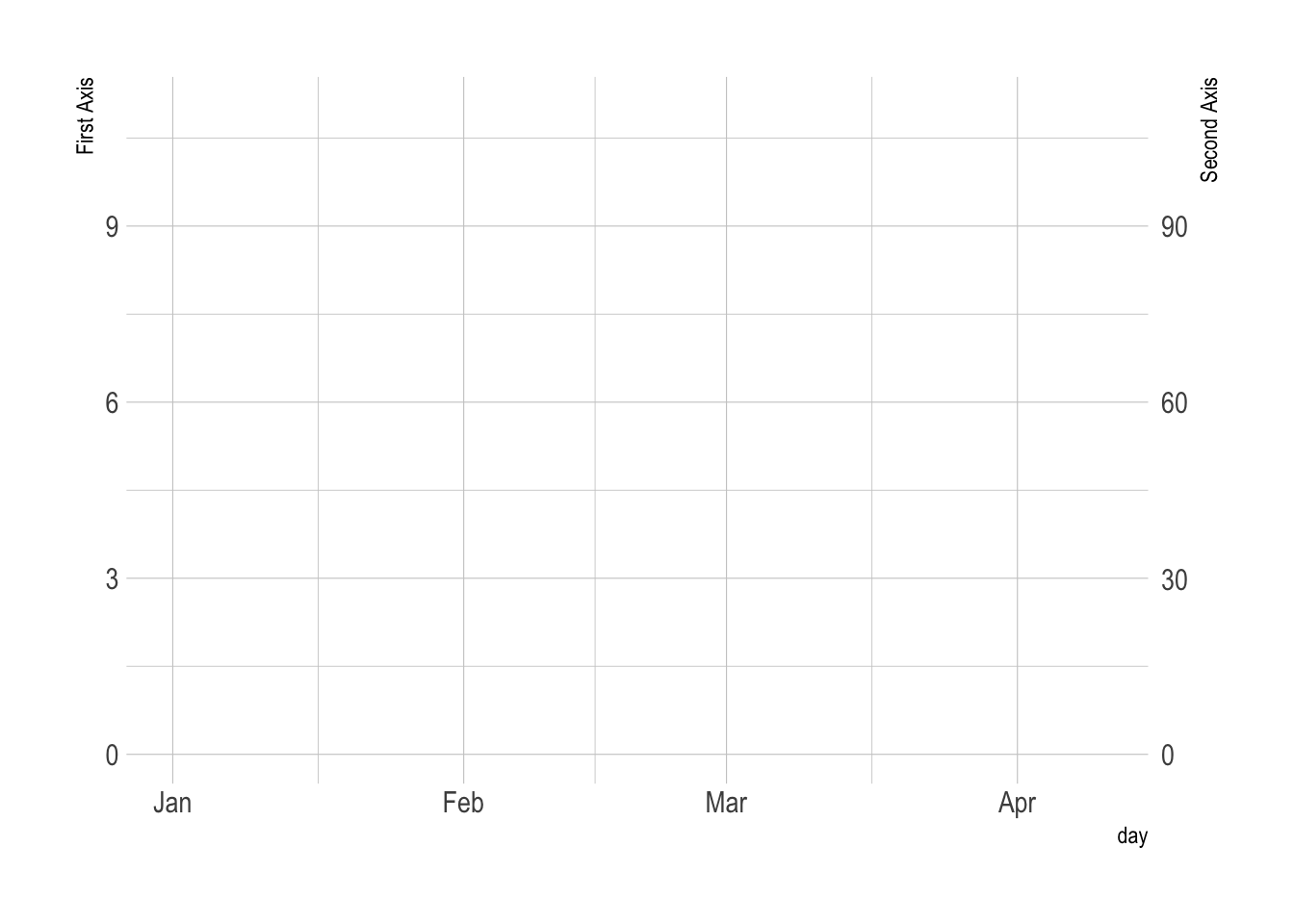``````# Start with a usual ggplot2 call:
ggplot(data, aes(x=day, y=temperature)) +

# Custom the Y scales:
scale_y_continuous(

# Features of the first axis
name = "First Axis",

# Add a second axis and specify its features
sec.axis = sec_axis( trans=~.*10, name="Second Axis")
) +

theme_ipsum()``````

# Show 2 series on the same line chart thanks to `sec.axis()`

We can use this `sec.axis` mathematical transformation to display 2 series that have a different range.

Since the price has a maximum value that is 10 times biggeer than the maximum temperature:

• the second Y axis is like the first multiplied by 10 (`trans=~.*10`).
• the value be display in the second variable `geom_line()` call must be divided by 10 to mimic the range of the first variable.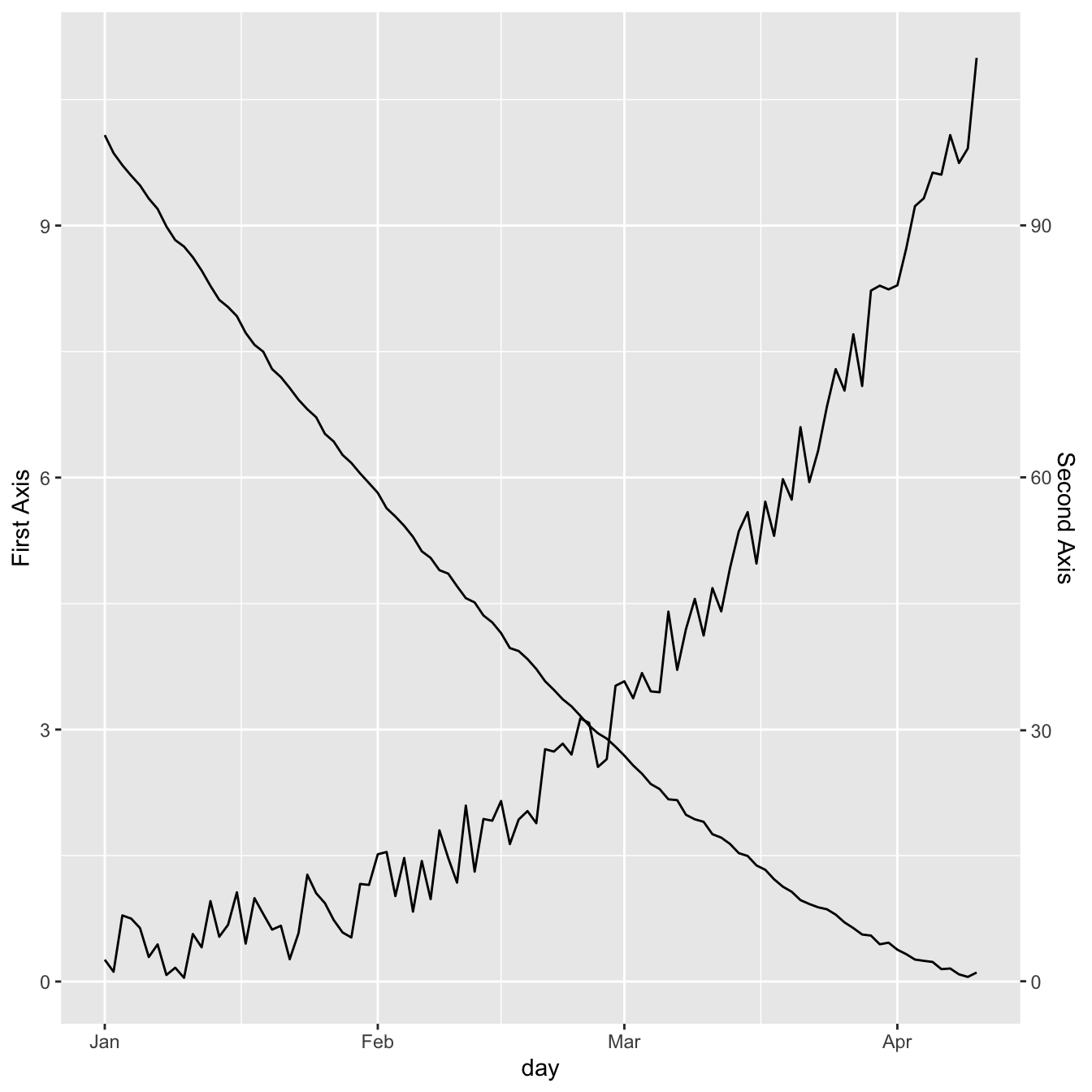``````# Value used to transform the data
coeff <- 10

ggplot(data, aes(x=day)) +

geom_line( aes(y=temperature)) +
geom_line( aes(y=price / coeff)) + # Divide by 10 to get the same range than the temperature

scale_y_continuous(

# Features of the first axis
name = "First Axis",

# Add a second axis and specify its features
sec.axis = sec_axis(~.*coeff, name="Second Axis")
)``````

# Dual Y axis customization with `ggplot2`

A feew usual tricks to make the chart looks better:

• `ipsum` theme to remove the black background and improve the general style
• customize the Y axes to pair them with their related line.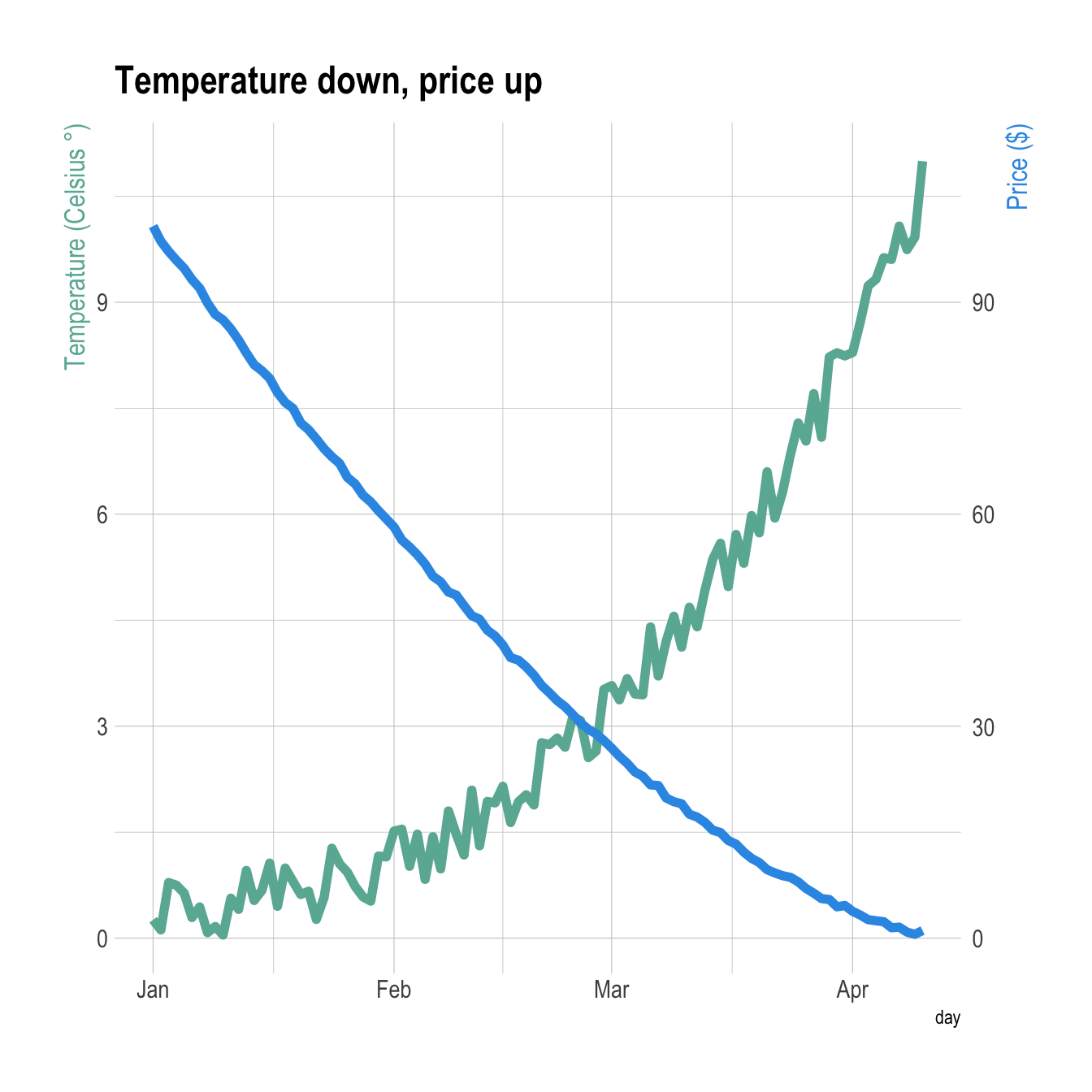``````# Value used to transform the data
coeff <- 10

# A few constants
temperatureColor <- "#69b3a2"
priceColor <- rgb(0.2, 0.6, 0.9, 1)

ggplot(data, aes(x=day)) +

geom_line( aes(y=temperature), size=2, color=temperatureColor) +
geom_line( aes(y=price / coeff), size=2, color=priceColor) +

scale_y_continuous(

# Features of the first axis
name = "Temperature (Celsius °)",

# Add a second axis and specify its features
sec.axis = sec_axis(~.*coeff, name="Price (\$)")
) +

theme_ipsum() +

theme(
axis.title.y = element_text(color = temperatureColor, size=13),
axis.title.y.right = element_text(color = priceColor, size=13)
) +

ggtitle("Temperature down, price up")``````

# Barplot with overlapping line chart

It is totally possible to usee the same tricks with other `geoms`.

Here is an example displaying a line chart on top of a barplot.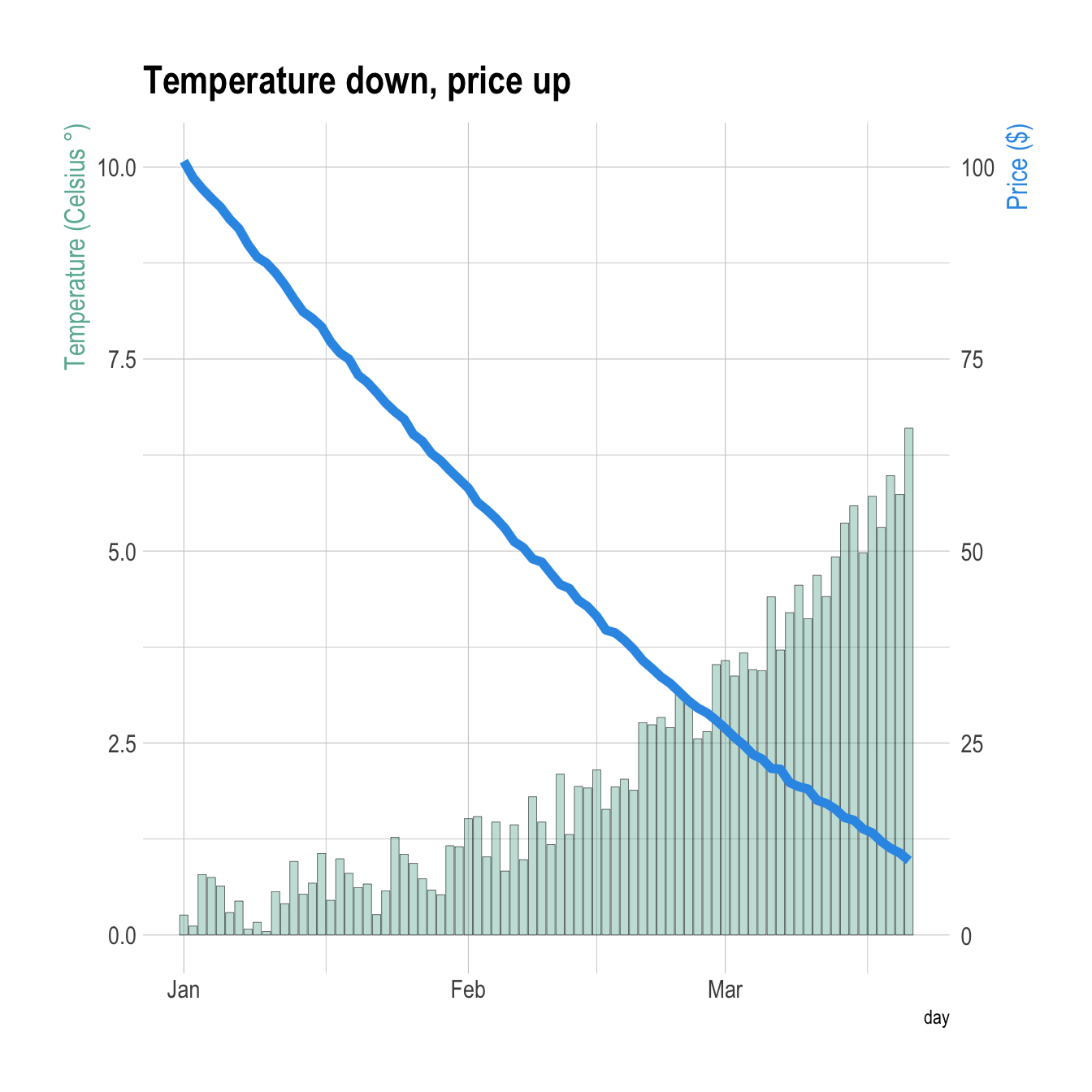``````# Value used to transform the data
coeff <- 10

# A few constants
temperatureColor <- "#69b3a2"
priceColor <- rgb(0.2, 0.6, 0.9, 1)

geom_bar( aes(y=temperature), stat="identity", size=.1, fill=temperatureColor, color="black", alpha=.4) +
geom_line( aes(y=price / coeff), size=2, color=priceColor) +

scale_y_continuous(

# Features of the first axis
name = "Temperature (Celsius °)",

# Add a second axis and specify its features
sec.axis = sec_axis(~.*coeff, name="Price (\$)")
) +

theme_ipsum() +

theme(
axis.title.y = element_text(color = temperatureColor, size=13),
axis.title.y.right = element_text(color = priceColor, size=13)
) +

ggtitle("Temperature down, price up")``````

Related chart types

## Contact

This document is a work by Yan Holtz. Any feedback is highly encouraged. You can fill an issue on Github, drop me a message on Twitter, or send an email pasting yan.holtz.data with gmail.com.